# Allometries and conversions

#### 2022-09-20

# Load TrenchR package

library(TrenchR)

This tutorial reviews tools that use allometries to estimate the dimensions of organisms needed for energy balances and other analyses. The tutorial also documents convenience functions for converting units. Finally, we present functions that describe thermal performance curves.

# Allometries

We provide tools for estimating additional dimensions of organisms from measured dimensions. ### Mass

The function mass_from_length() encompasses empirically-derived relationships to estimate mass as a power law function of mass for six taxonomic classifications. The relationships are derived by relating length to mass for specimens of numerous species. We use the mass_from_length() function to illustrate the allometries for different taxa:

oldpar<- par()

par(mar = c(5, 5, 2, 2))

plot(x    = seq(0.01, 0.25, 0.01),
y    = mass_from_length(l     = seq(0.01, 0.25, 0.01),
taxon = "lizard"),
type = "l",
xlab = "length (m)",
ylab = "mass (g)",
ylim = c(0, 200),
xlim = c(0, 0.25),
las  = 1,
bty  = "L",
lwd  = 2)

points(x    = seq(0.01, 0.25, 0.01),
y    = mass_from_length(l     = seq(0.01, 0.25, 0.01),
taxon = "salamander"),
type = "l",
col  = "blue",
lwd  = 2)

points(x    = seq(0.01, 0.25, 0.01),
y    = mass_from_length(l     = seq(0.01, 0.25, 0.01),
taxon = "frog"),
type = "l",
col  = "red",
lwd  = 2)

points(x    = seq(0.01, 0.25, 0.01),
y    = mass_from_length(l     = seq(0.01, 0.25, 0.01),
taxon = "snake"),
type = "l",
col  = "green",
lwd  = 2)

points(x    = seq(0.01, 0.25, 0.01),
y    = mass_from_length(l     = seq(0.01, 0.25, 0.01),
taxon = "turtle"),
type = "l",
col  = "orange",
lwd  = 2)

points(x    = seq(0.01, 0.25, 0.01),
y    = mass_from_length(l     = seq(0.01, 0.25, 0.01),
taxon = "insect"),
type = "l",
col  = "purple",
lwd  = 2)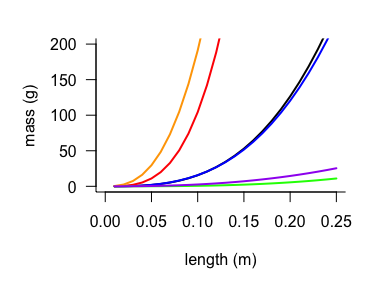### Surface Area

Surface area is needed for several aspects of biophysical models but is rarely measured. We provide functions to estimate surface area ($$m^3$$) from length, mass, or volume for a variety of taxa.

The function surface_area_from_mass() encompasses empirically-derived relationship to estimate surface area as a power law function of mass for lizards, frogs, and insects.

par(mar = c(5, 5, 2, 2))

plot(x    = 1:50,
y    = surface_area_from_mass(m     = 1:50,
taxon = "lizard"),
type = "l",
xlab = "mass (g)",
ylab = "",
las  = 1,
bty  = "L",
lwd  = 2)

mtext(text = expression("surface area" ~ (m^{2})),
side = 2,
line = 3.5)

points(x    = 1:50,
y    = surface_area_from_mass(m     = 1:50,
taxon = "frog"),
type = "l",
lty  = "dashed",
lwd  = 2)

points(x    = 1:50,
y    = surface_area_from_mass(m     = 1:50,
taxon = "salamander"),
type = "l",
lty  = "dotted",
lwd  = 2)
points(x    = seq(0.1, 5, 0.2),
y    = surface_area_from_mass(m     = seq(0.1,5,0.2),
taxon = "insect"),
type = "l",
lty  = "dotdash",
lwd   = 2)

box(bty = "L",
lwd = 2)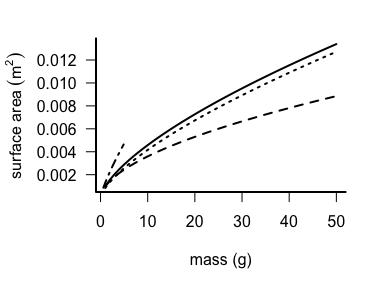We also provide empirical relationships estimating surface area as power law function of volume. The functions were developed by Mitchell (1976) for use in approximating convective heat transfer:

plot(x    = seq(0.001, 0.01, 0.001),
y    = surface_area_from_volume(V     = seq(0.001, 0.01, 0.001),
taxon = "lizard"),
type = "l",
xlab = expression(volume ~ (m^{3})),
ylab = expression("surface area" ~ (m^{2})))

points(x    = seq(0.001, 0.01, 0.001),
y    = surface_area_from_volume(V     = seq(0.001, 0.01, 0.001),
taxon = "frog"),
type = "l",
lty  = "dashed")

points(x    = seq(0.001, 0.01, 0.001),
y    = surface_area_from_volume(V     = seq(0.001, 0.01, 0.001),
taxon = "sphere"),
type = "l",
lty  = "dotted")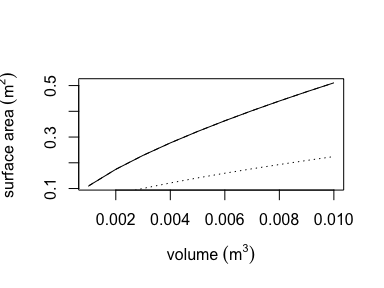For the same study, we additionally provide a function to estimate volume from length for several taxa or by approximating the organism as a sphere. Finally, we provide a function for estimating surface area from length by approximating the animal’s body as a rotational ellipsoid. The allometric functions are available in R as follows:

par(mar = c(5, 5, 2, 2))

plot(x    = seq(0.01, 0.05, 0.001),
y    = volume_from_length(l     = seq(0.01, 0.05, 0.001),
taxon = "lizard"),
type = "l",
xlab = "length (m)",
ylab = expression(volume ~ (m^{3})))

points(x    = seq(0.01, 0.05, 0.001),
y    = volume_from_length(l     = seq(0.01, 0.05, 0.001),
taxon = "frog"),
type = "l",
lty  = "dashed")

points(x    = seq(0.01, 0.05, 0.001),
y    = volume_from_length(l     = seq(0.01, 0.05, 0.001),
taxon = "sphere"),
type = "l",
lty  = "dotted")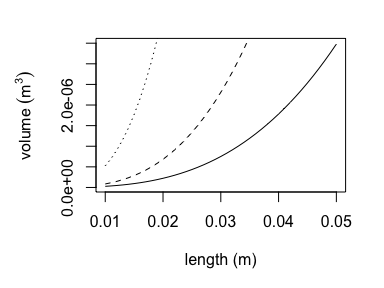par(mar = c(5, 5, 2, 2))

plot(x    = seq(0.01, 0.05, 0.001),
y    = surface_area_from_length(l = seq(0.01, 0.05, 0.001)),
type = "l",
xlab = "length (m)",
ylab = expression("surface area" ~ (m^{2})))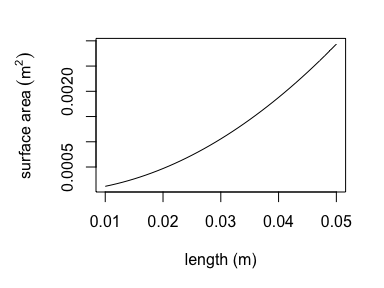Estimating the amount of solar radiation that an animal absorbs requires knowledge of the silhouette area, the proportion of an animal’s surface area that is projected onto a horizontal surface. Silhouette area is a function of the sun’s angle. We offer empirically-derived relationships to predict the proportional silhouette area for frogs, lizards, and grasshoppers as a polynomial function of zenith angle (proportion_silhouette_area()). We additionally offer a function (proportion_silhouette_area_shapes()) to estimate silhouette area by approximating the animal as a sphere or cylinder as a function of theta, the angle between the solar beam and the longitudinal axis. The R functions are parameterized as follows:

par(mar = c(5, 5, 2, 2))

plot(x    = seq(0, 90, 10),
y    = proportion_silhouette_area(z     = seq(0, 90, 10),
taxon = "frog"),
type = "l",
xlab = "zenith angle (°)",
ylab = "proportion silhouette area",
ylim = range(0, 0.5))

points(x    = seq(0, 90, 10),
y    = proportion_silhouette_area(z       = seq(0, 90, 10),
taxon   = "lizard",
raz     = 0,
posture = "prostrate"),
type = "l",
lty  = "dashed")

points(x    = seq(0, 90, 10),
y    = proportion_silhouette_area(z       = seq(0, 90, 10),
taxon   = "lizard",
raz     = 0,
posture = "elevated"),
type = "l",
lty  = "dotted")
points(x    = seq(0, 90, 10),
y    = proportion_silhouette_area(z     = seq(0, 90, 10),
taxon =  "grasshopper"),
type = "l",
lty  = "dotdash")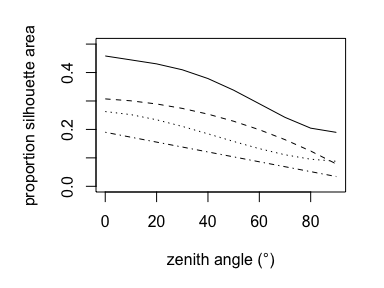plot(x    = seq(0, 90, 10),
y    = proportion_silhouette_area_shapes(shape = "spheroid",
theta = seq(0, 90, 10),
h     = 0.4,
d     = 0.39),
type = "l",
xlab = "theta (°)",
ylab = "proportion silhouette area",
ylim = c(0, 0.35),
lty  = "dashed")

points(x    = seq(0, 90, 10),
y    = proportion_silhouette_area_shapes(shape = "spheroid",
theta = seq(0, 90, 10),
h     = 0.4,
d     = 0.3),
type = "l",
lty  = "dashed")

points(x    = seq(0, 90, 10),
y    = proportion_silhouette_area_shapes(shape = "spheroid",
theta = seq(0, 90, 10),
h     = 0.4,
d     = 0.2),
type = "l",
lty  = "dotted")

points(x    = seq(0, 90, 10),
y    = proportion_silhouette_area_shapes(shape = "spheroid",
theta = seq(0, 90, 10),
h     = 0.4,
d     = 0.1),
type = "l",
lty  = "dotdash")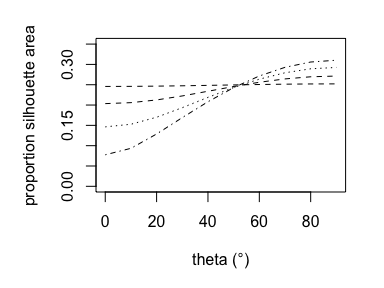plot(x    = seq(0, 90, 10),
y    = proportion_silhouette_area_shapes(shape = "cylinder flat ends",
theta = seq(0, 90, 10),
h     = 0.4,
d     = 0.4),
type = "l",
lty  = "dashed",
xlab = "theta (°)",
ylab = "proportion silhouette area",
ylim = c(0, 0.35))

points(x    = seq(0, 90, 10),
y    = proportion_silhouette_area_shapes(shape = "cylinder flat ends",
theta = seq(0, 90, 10),
h     = 0.4,
d     = 0.2),
type = "l",
lty  = "dashed")

points(x    = seq(0, 90, 10),
y    = proportion_silhouette_area_shapes(shape = "cylinder flat ends",
theta = seq(0, 90, 10),
h     = 0.4,
d     = 0.1),
type = "l",
lty  = "dotted")

points(x    = seq(0, 90, 10),
y    = proportion_silhouette_area_shapes(shape = "cylinder flat ends",
theta = seq(0, 90, 10),
h     = 0.4,
d     = 0.05),
type = "l",
lty  = "dotdash")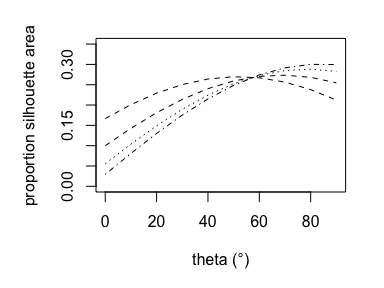plot(x    = seq(0, 90, 10),
y    = proportion_silhouette_area_shapes(shape = "cylinder hemisphere ends",
theta = seq(0, 90, 10),
h     = 0.4,
d     = 0.4),
type = "l",
xlab = "theta (°)",
ylab = "proportion silhouette area",
ylim = c(0, 0.35))

points(x    = seq(0, 90, 10),
y    = proportion_silhouette_area_shapes(shape = "cylinder hemisphere ends",
theta = seq(0, 90, 10),
h     = 0.4,
d     = 0.2),
type = "l",
lty  = "dashed")

points(x    = seq(0, 90, 10),
y    = proportion_silhouette_area_shapes(shape = "cylinder hemisphere ends",
theta = seq(0, 90, 10),
h     = 0.4,
d     = 0.1),
type = "l",
lty  = "dotted")

points(x    = seq(0, 90, 10),
y    = proportion_silhouette_area_shapes(shape = "cylinder hemisphere ends",
theta = seq(0, 90, 10),
h     = 0.4,
d     = 0.05),
type = "l",
lty  = "dotdash")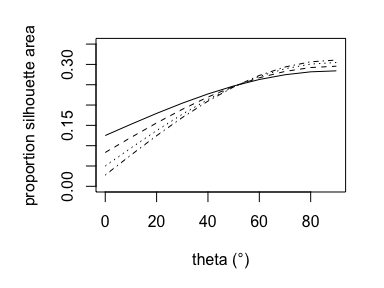# Conversions

We offer convenience functions to convert among units for angles and temperatures:

degrees_to_radians(47.608)
##  0.8309164
radians_to_degrees(0.831)
##  47.61279
fahrenheit_to_celsius(85)
##  29.44444
fahrenheit_to_kelvin(85)
##  302.5944
kelvin_to_celsius(270)
##  -3.15

# Thermal Performance Curve Functions

Thermal performance curves (TPCs) describe the temperature dependence of organismal performance such as locomotion and digestion (Huey and Kingsolver 1989). They have become an important tool to understand organismal responses to environmental conditions, particularly responses to climate change (Sinclair et al. 2016). TrenchR includes two forms of TPCs. We include a function TPC() to describe a Gaussian-quadratic TPC as a function of thermal optima and critical thermal minima and maxima (Deutsch et al. 2008). The TPC_beta() function uses a beta function to define a TPC based on mode, breadth, and skew. A scale factor enables including a thermodynamic effect on mean performance (Asbury and Angilletta Jr 2010). Additional TPC resources are available in the R packages rTPC and thermPref.

plot(x    = 0:60,
y    = TPC(T      = 0:60,
T_opt  = 30,
CT_min = 10,
CT_max = 40),
type = "l",
ylim = c(0,3),
ylab = "performance",
xlab = "temperature (°C)")

points(x    = 0:60,
y    = TPC_beta(T         = 0:60,
shift     = -1,
aran      = 0,
tolerance = 43,
skew      = 0.7),
type = "l",
lty  = "dashed")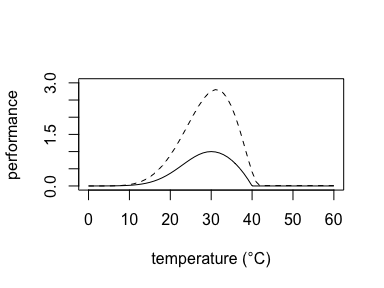par(oldpar)
## Warning in par(oldpar): graphical parameter "cin" cannot be set
## Warning in par(oldpar): graphical parameter "cra" cannot be set
## Warning in par(oldpar): graphical parameter "csi" cannot be set
## Warning in par(oldpar): graphical parameter "cxy" cannot be set
## Warning in par(oldpar): graphical parameter "din" cannot be set
## Warning in par(oldpar): graphical parameter "page" cannot be set